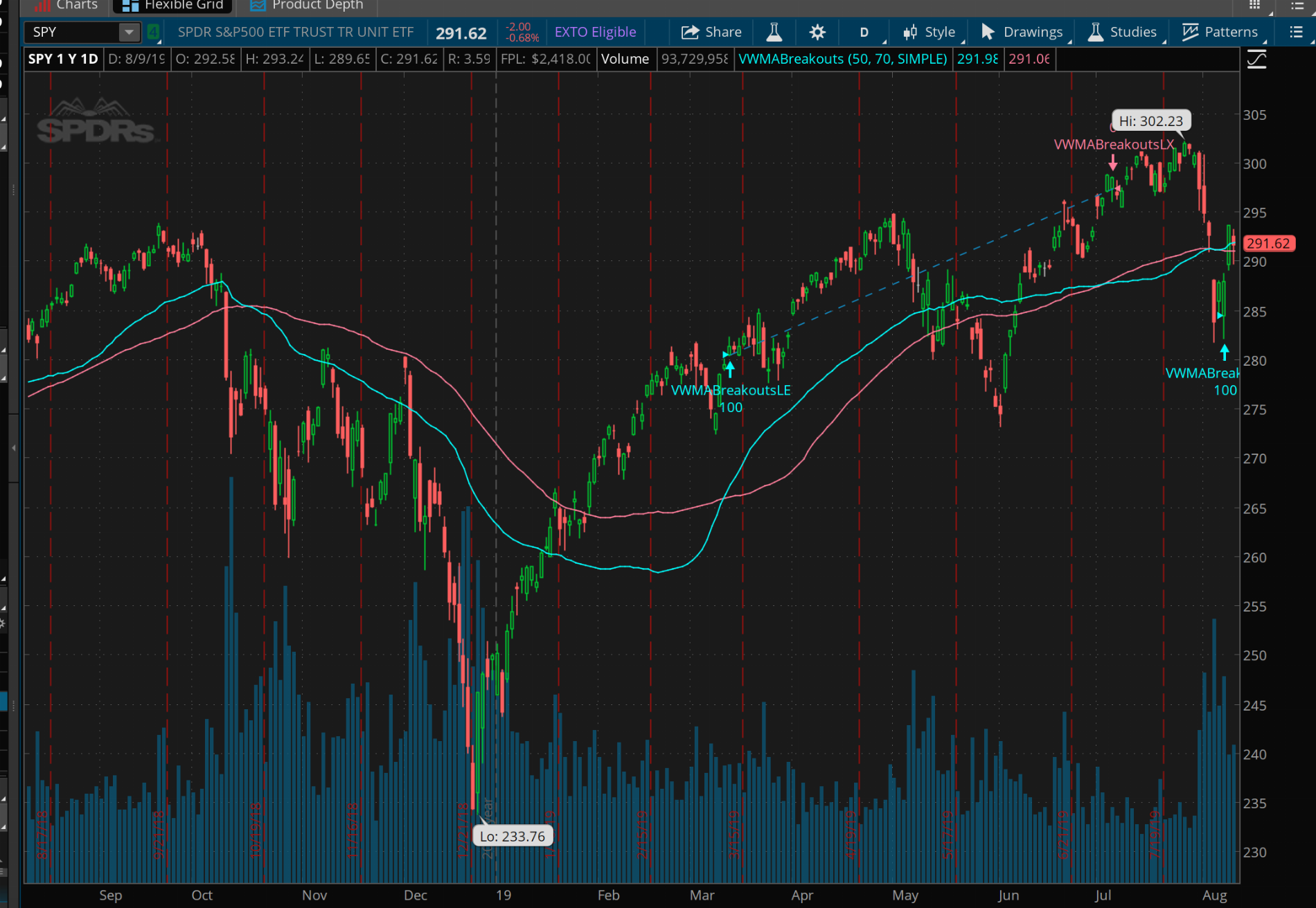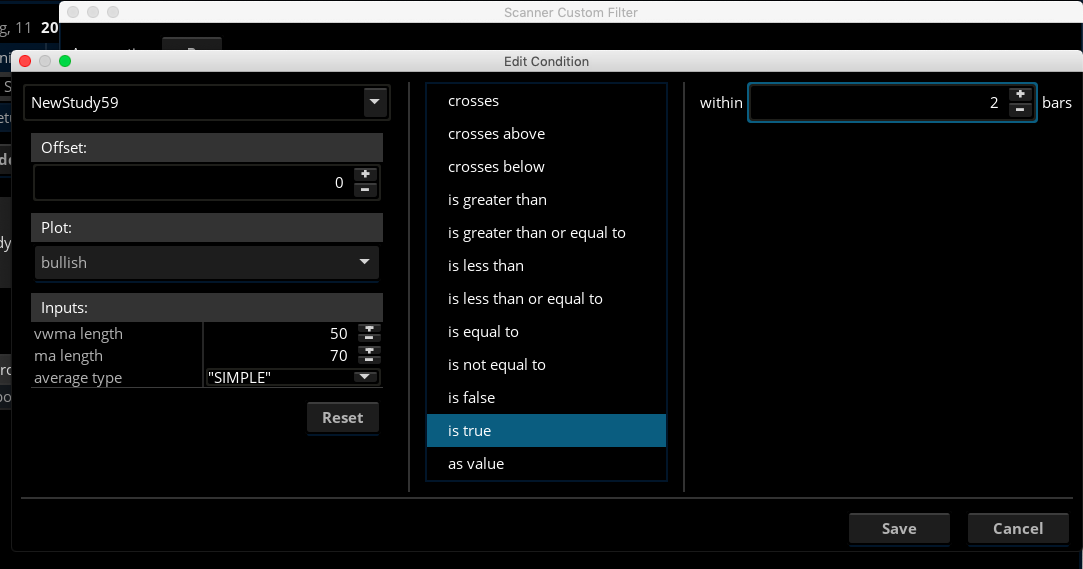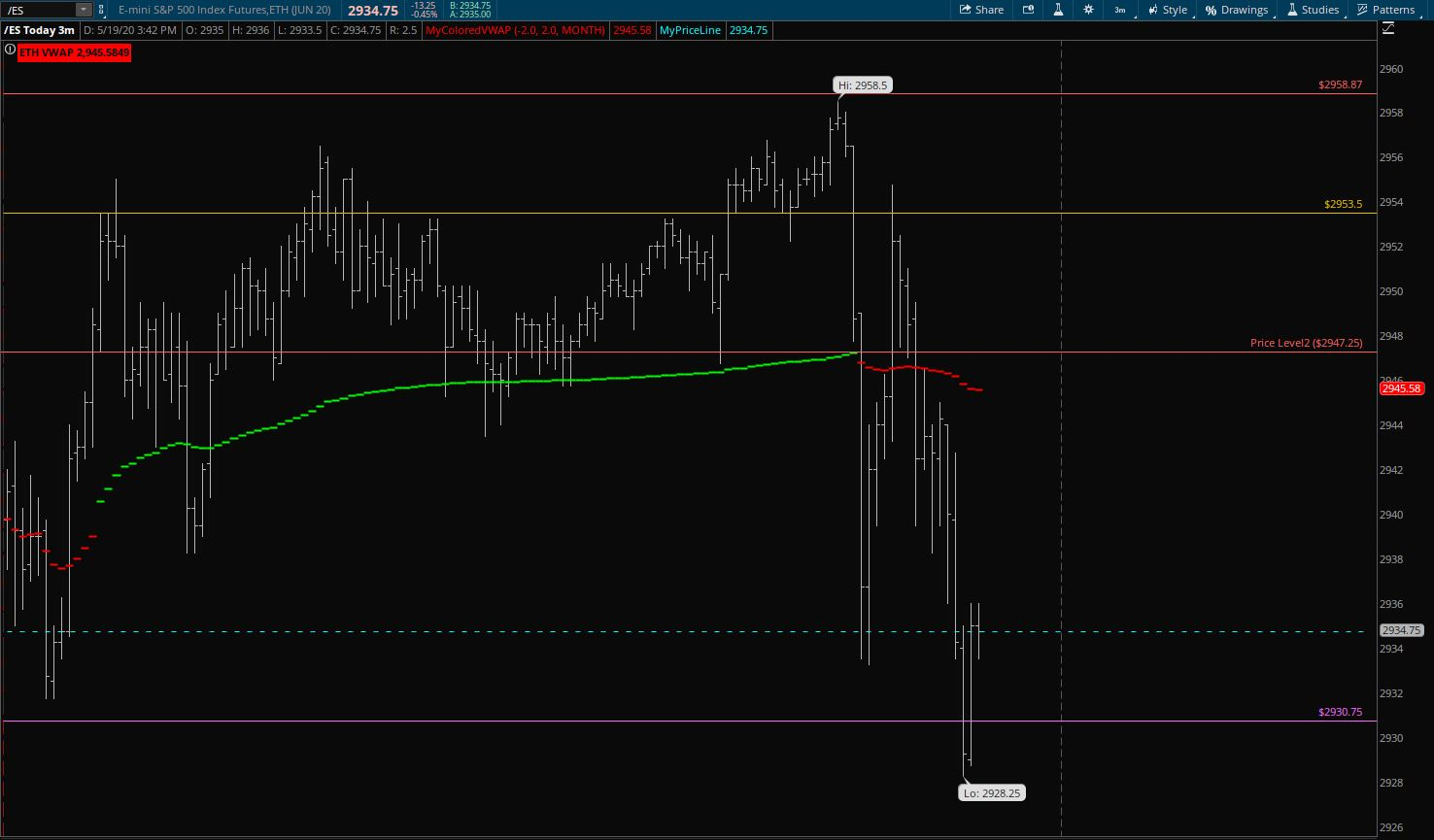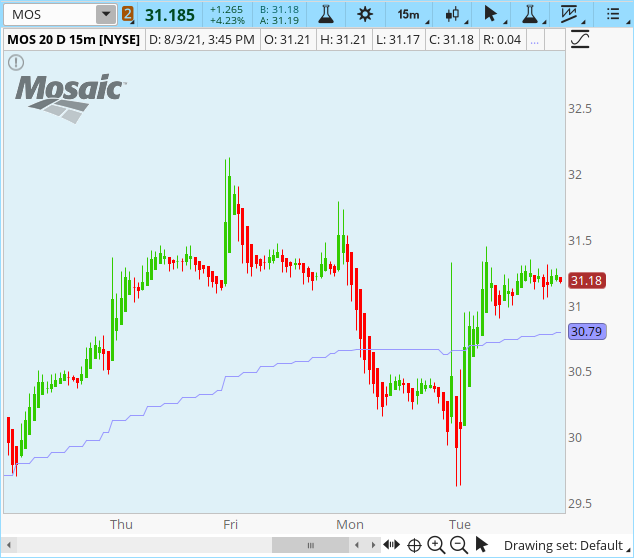# Volume Weighted (VWMA) Breakout for ThinkorSwim

#### veerasareddy

##### New member
Could you please help me to give the scanner for VWMA Breakout strategy in ThinkorSwim. When the Blue line crosses above or below the Pink line in Daily / 4h Chart.Thank you.

#### Attachments

Here you go. Save this as a study. Then switch over to the Scanner and scan for buy and sell signals.

Code:
``````# VWMA Breakout Strategy
# TD Ameritrade IP Company, Inc. (c) 2017-2019
# Modified by BenTen at useThinkScript.com
# Added arrows to use with the Scanner

input vwmaLength = 50;
input maLength = 70;
input averageType = AverageType.SIMPLE;

plot VWMA = Sum(volume * close, vwmaLength) / Sum(volume, vwmaLength);
plot MA = MovingAverage(averageType, close, maLength);
VWMA.SetDefaultColor(GetColor(1));
MA.SetDefaultColor(GetColor(2));

def buy = VWMA crosses above MA;
def sell = VWMA crosses below MA;

bullish.SetPaintingStrategy(PaintingStrategy.BOOLEAN_Arrow_UP);
bullish.SetDefaultColor(Color.LIME);
bullish.SetLineWeight(1);

plot bearish = sell;
bearish.SetPaintingStrategy(PaintingStrategy.BOOLEAN_Arrow_DOWN);
bearish.SetDefaultColor(Color.LIME);
bearish.SetLineWeight(1);``````

•Magic and Zachc
@BenTen Thank you so much. I saved as a study. But I'm not able to scan BUY / SELL as per your instructions. Could you please help one more step. Thank you for your kindness. Probably a screen shot of the scanner code.

Code:
``````MovAvgWeighted("length" = 50) crosses above SimpleMovingAverage("length" = 70) within 3 bars
MovAvgWeighted("length" = 50) crosses below SimpleMovingAverage("length" = 70) within 3 bars``````

Just try scan similiar to above , Do not have ToS open so unsure if the "SimpleMovingAverage" is the correct spelling for that study. If not just look in studies for the correct wording.

@veerasareddy Switch to Scan tab, Add new filter study > Select the study I sent you above > Under Plot select the type of signal you want to scan for and also within how many bars.Last edited:
•Magic
Folks, I looking for the VWMA. I have searched far and wide, but can't find the code or link for it, only lots of talk about how it works.

This is not the VWMA Breakout, which is already part of ThinkorSwim.

WayneG

Do you mean VWAP?Last edited:
No, it is the Volume Weighted Moving Average (VWMA), the VWAP throws price into the mix.

No, it is the Volume Weighted Moving Average (VWMA), the VWAP throws price into the mix.

Volume Weighted Moving Average

The Volume Weighted Moving Average (VWMA) study calculates the average weighted price by volume over a period of N bars. The formula is as follows: SUM(vol*price)/SUM(vol). The user may change the input (close), period and shift.

Code:
``````input LENGTH = 5;
input displace = 0;

def VWMA = Sum(volume * close) / Sum(volume);
plot MYVWMA = Average(VWMA[-displace], LENGTH);``````

•BillW
TOS MTF VWMA , can it be done?

mtf VWMA (multi-timeframe Volume Weighted Moving Average)
The key differences between VWAP and VWMA are: VWAP is cumulative of average price with respect to volume. ... VWMA is a type of moving averages. The indicator calculates the average of closing prices with respect to the volume.

This was a poster request. I haven't traded with this indicator personally. If you use it, please leave feedback.Ruby:
``````# ########################################################
# VWMA MTF
# @MerryDay 7/2021
# ########################################################
# MTF definitions
input agg = aggregationPeriod.HOUR ;
def mtf_volume = volume(period = agg) ;
def mtf_price  = close(period = agg) ;
# ########################################################

input vwmaLength = 50;
input averageType = AverageType.SIMPLE;
plot VWMA = Sum(mtf_volume * mtf_price, vwmaLength) / Sum(mtf_volume, vwmaLength);
VWMA.SetDefaultColor(color.violet) ;``````

I was reading about volume-weighted moving average and this was what I could come up with. I noticed that when I used the simple moving average for shorter periods the value would be quite low, so I calculated the exponential moving average instead, also when I calculated the 50 periods it would vary widely on the chart, I am not sure if I made a mistake in the code. I am hoping someone could possibly simplify/audit the codes

10 Period Volume Weighted Exponential Moving Average
Ruby:
``````def total_volume = sum(volume,10);
def vw = (volume/total_volume);
def vw2 = (volume()/total_volume);
def vw3 = (volume()/total_volume);
def vw4 = (volume()/total_volume);
def vw5 = (volume()/total_volume);
def vw6 = (volume()/total_volume);
def vw7 = (volume()/total_volume);
def vw8 = (volume()/total_volume);
def vw9 = (volume()/total_volume);
def vw10 = (volume()/total_volume);
def vwp = close * vw;
def vwp2 = close * vw2;
def vwp3 = close * vw3;
def vwp4 = close * vw4;
def vwp5 = close * vw5;
def vwp6 = close * vw6;
def vwp7 = close * vw7;
def vwp8 = close * vw8;
def vwp9 = close * vw9;
def vwp10 = close* vw10;

plot ema = expaverage(vwp + vwp2 + vwp3 + vwp4 + vwp5 + vwp6 + vwp7 + vwp8 + vwp9 + vwp10 );

ema.SetDefaultColor(GetColor(1));``````

20 Period Volume Weighted Exponential Moving Average
Code:
``````def total_volume = sum(volume,20);
def vw = (volume/total_volume);
def vw2 = (volume()/total_volume);
def vw3 = (volume()/total_volume);
def vw4 = (volume()/total_volume);
def vw5 = (volume()/total_volume);
def vw6 = (volume()/total_volume);
def vw7 = (volume()/total_volume);
def vw8 = (volume()/total_volume);
def vw9 = (volume()/total_volume);
def vw10 = (volume()/total_volume);
def vwp = close * vw;
def vwp2 = close * vw2;
def vwp3 = close * vw3;
def vwp4 = close * vw4;
def vwp5 = close * vw5;
def vwp6 = close * vw6;
def vwp7 = close * vw7;
def vwp8 = close * vw8;
def vwp9 = close * vw9;
def vwp10 = close* vw10;
def vw11 = (volume/total_volume);
def vw12 = (volume()/total_volume);
def vw13 = (volume()/total_volume);
def vw14 = (volume()/total_volume);
def vw15 = (volume()/total_volume);
def vw16 = (volume()/total_volume);
def vw17 = (volume()/total_volume);
def vw18 = (volume()/total_volume);
def vw19 = (volume()/total_volume);
def vw20 = (volume()/total_volume);
def vwp11 = close * vw11;
def vwp12 = close * vw12;
def vwp13 = close * vw13;
def vwp14 = close * vw14;
def vwp15 = close * vw15;
def vwp16 = close * vw16;
def vwp17 = close * vw17;
def vwp18 = close * vw18;
def vwp19 = close * vw19;
def vwp20 = close*  vw20;
plot ema = expaverage(vwp + vwp2 + vwp3 + vwp4 + vwp5 + vwp6 + vwp7 + vwp8 + vwp9 + vwp10 + vwp11 + vwp12 + vwp13 + vwp14 + vwp15 + vwp16 + vwp17 + vwp18 + vwp19 +vwp20 );

ema.SetDefaultColor(GetColor(1));``````

50 Period Volume Weighted Simple Moving Average
Code:
``````def total_volume = sum(volume,50);
def vw = (volume/total_volume);
def vw2 = (volume()/total_volume);
def vw3 = (volume()/total_volume);
def vw4 = (volume()/total_volume);
def vw5 = (volume()/total_volume);
def vw6 = (volume()/total_volume);
def vw7 = (volume()/total_volume);
def vw8 = (volume()/total_volume);
def vw9 = (volume()/total_volume);
def vw10 = (volume()/total_volume);
def vwp = close * vw;
def vwp2 = close * vw2;
def vwp3 = close * vw3;
def vwp4 = close * vw4;
def vwp5 = close * vw5;
def vwp6 = close * vw6;
def vwp7 = close * vw7;
def vwp8 = close * vw8;
def vwp9 = close * vw9;
def vwp10 = close* vw10;
def vw11 = (volume()/total_volume);
def vw12 = (volume()/total_volume);
def vw13 = (volume()/total_volume);
def vw14 = (volume()/total_volume);
def vw15 = (volume()/total_volume);
def vw16 = (volume()/total_volume);
def vw17 = (volume()/total_volume);
def vw18 = (volume()/total_volume);
def vw19 = (volume()/total_volume);
def vw20 = (volume()/total_volume);
def vwp11 = close * vw11;
def vwp12 = close * vw12;
def vwp13 = close * vw13;
def vwp14 = close * vw14;
def vwp15 = close * vw15;
def vwp16 = close * vw16;
def vwp17 = close * vw17;
def vwp18 = close * vw18;
def vwp19 = close * vw19;
def vwp20 = close *  vw20;
def vw21 = (volume()/total_volume);
def vw22 = (volume()/total_volume);
def vw23 = (volume()/total_volume);
def vw24 = (volume()/total_volume);
def vw25 = (volume()/total_volume);
def vw26 = (volume()/total_volume);
def vw27 = (volume()/total_volume);
def vw28 = (volume()/total_volume);
def vw29 = (volume()/total_volume);
def vw30 = (volume()/total_volume);
def vwp21 = close * vw;
def vwp22 = close * vw2;
def vwp23 = close * vw3;
def vwp24 = close * vw4;
def vwp25 = close * vw5;
def vwp26 = close * vw6;
def vwp27 = close * vw7;
def vwp28 = close * vw8;
def vwp29 = close * vw9;
def vwp30 = close * vw10;
def vw31 = (volume()/total_volume);
def vw32 = (volume()/total_volume);
def vw33 = (volume()/total_volume);
def vw34 = (volume()/total_volume);
def vw35 = (volume()/total_volume);
def vw36 = (volume()/total_volume);
def vw37 = (volume()/total_volume);
def vw38 = (volume()/total_volume);
def vw39 = (volume()/total_volume);
def vw40 = (volume()/total_volume);
def vwp31 = close * vw31;
def vwp32 = close * vw32;
def vwp33 = close * vw33;
def vwp34 = close * vw34;
def vwp35 = close * vw35;
def vwp36 = close * vw36;
def vwp37 = close * vw37;
def vwp38 = close * vw38;
def vwp39 = close * vw39;
def vwp40 = close * vw40;
def vw41 = (volume()/total_volume);
def vw42 = (volume()/total_volume);
def vw43 = (volume()/total_volume);
def vw44 = (volume()/total_volume);
def vw45 = (volume()/total_volume);
def vw46 = (volume()/total_volume);
def vw47 = (volume()/total_volume);
def vw48 = (volume()/total_volume);
def vw49 = (volume()/total_volume);
def vw50 = (volume()/total_volume);
def vwp41 = close * vw41;
def vwp42 = close * vw42;
def vwp43 = close * vw43;
def vwp44 = close * vw44;
def vwp45 = close * vw45;
def vwp46 = close * vw46;
def vwp47 = close * vw47;
def vwp48 = close * vw48;
def vwp49 = close * vw49;
def vwp50 = close * vw50;
plot sma = average(vwp + vwp2 + vwp3 + vwp4 + vwp5 + vwp6 + vwp7 + vwp8 + vwp9 + vwp10 + vwp11 + vwp12 + vwp13 + vwp14 + vwp15 +vwp16 + vwp17 + vwp18 +vwp19 +vwp20 +vwp21  + vwp22 + vwp23 + vwp24 + vwp25 + vwp26 +vwp27 + vwp28 + vwp29 + vwp30 + vwp31 + vwp32 + vwp33 + vwp34 + vwp35 + vwp36 + vwp37 + vwp38 + vwp39 + vwp40 + vwp41 + vwp42 + vwp43 + vwp44 + vwp45 + vwp46 + vwp47 + vwp48 + vwp49 + vwp50 );

sma.SetDefaultColor(GetColor(1));``````
.

I was reading about volume-weighted moving average and this was what I could come up with. I noticed that when I used the simple moving average for shorter periods the value would be quite low, so I calculated the exponential moving average instead, also when I calculated the 50 periods it would vary widely on the chart, I am not sure if I made a mistake in the code. I am hoping someone could possibly simplify/audit the codes
.
I moved your post here. You will find the VWMA scripts in this thread, a wee bit more concise.

•ziongotoptions
Hello BenTen---Capt Bob here ---I like your study and I've incorporated another study to weed out the false signals and would like the code to the original Vwma ---I assume you wrote the original code or have it . I need it to automate my strat. Is this possible to obtain. Tks Capt Bob

Good evening Benten---This code seems to be locked can I get an unlocked version for my Strat. As I added another study to eliminate some false trades. Thanks Capt.Bob

Hello Benten---can you send me a version that eliminates the words on the chart. The code seems to be locked and all I need is arrows up or down. I'm new to TOS and as I said before I've got another study that will eliminate a lot of false trades but need the code to make it happen please advise if this is possible. Thanks Capt.Bob

Last edited by a moderator:
Hello BenTen---Capt Bob here ---I like your study and I've incorporated another study to weed out the false signals and would like the code to the original Vwma ---I assume you wrote the original code or have it . I need it to automate my strat. Is this possible to obtain. Tks Capt Bob

Good evening Benten---This code seems to be locked can I get an unlocked version for my Strat. As I added another study to eliminate some false trades. Thanks Capt.Bob

Hello Benten---can you send me a version that eliminates the words on the chart. The code seems to be locked and all I need is arrows up or down. I'm new to TOS and as I said before I've got another study that will eliminate a lot of false trades but need the code to make it happen please advise if this is possible. Thanks Capt.Bob
Not sure what you are asking. The script in the top post is not 'locked'

If you are asking about a ToS 'locked' study then you are correct. ToS copy-protected scripts can be used as is. But the ToS platform does not provide the code or editing / modifying capabilities for its copy-protected scripts.

### Not the exact question you're looking for?

87k+ Posts
233 Online## The Market Trading Game Changer

Join 2,500+ subscribers inside the useThinkScript VIP Membership Club
• Exclusive indicators
• Proven strategies & setups
• Private Discord community
• Exclusive members-only content
• 1 full year of unlimited support

What is useThinkScript?

useThinkScript is the #1 community of stock market investors using indicators and other tools to power their trading strategies. Traders of all skill levels use our forums to learn about scripting and indicators, help each other, and discover new ways to gain an edge in the markets.

How do I get started?

We get it. Our forum can be intimidating, if not overwhelming. With thousands of topics, tens of thousands of posts, our community has created an incredibly deep knowledge base for stock traders. No one can ever exhaust every resource provided on our site.

If you are new, or just looking for guidance, here are some helpful links to get you started.

What are the benefits of VIP Membership?